Ohm's Law

## A little bit of history...

Back in 1827 a great discovery was revealed by Georg Ohm.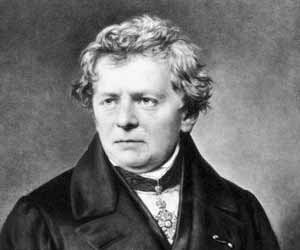Georg Simon Ohm (1787-1854) was a German physicist (electrician) and mathematician. Making experiments and using his equipment,
he realized that different conductive materials he used, showed him that current gets through them with different difficulty according to
the potential difference. (Voltage).
In other words, the current in a conductor is directly proportional to the voltage and inversely proportional to the resistance.
he well known Ohm's Law. The name was given as a memorial to Georg Ohm discovery.

Below it's Ohm's life timeline. It was taken from thefamouspeople.com (Click on it to see his full biography)

Georg Simon Ohm (1787-1854)

1787: Georg Simon Ohm was born
1800: Was enrolled in Erlangen Gymnasium
1805: Took aadmission in the University of Erlangen
1806: Was sent to Switzerland; Took up a post as a mathematics teacher in a school in Gottstadt bei Nydau
1809: Became a private tutor in NeuchActel
1811: Returned to the University of Erlangen; Received his PhD degree from the University of Erlangen
1813: Took up the position of a teacher in mathematics and physics in Bamberg
1817: Offered a position of a teacher in mathematics and physics at the Jesuit Gymnasium of Cologne.
1825: Submitted his first paper
1826: Presented two more papers; Was given a year off by the Jesuit Gymnasium of Cologne
1827: Published his famous book, Die galvanische Kette; Resumed work in the Jesuit Gymnasium of Cologne
1828: Resigned his position
1833: Ohm accepted the position of a professor at the Nuremburg
1842: Received the Copley Medal award by the Royal Society
1843: Appointed as the foreign member of the Royal Society
1845: Became a full member of the Bavarian Academy.
1849: Took up a post in Munich as a curator of the Bavarian Academy's physics cabinet
1852: Offered the chair of physics at the University of Munich
1854: Georg Simon Ohm breathed his last

## Ohm's Law analysis... The Ohm's triangle

Ok... let's go now more deeper to analyze the Ohms Law.
In circuit analysis, three equivalent expressions of Ohm's law are used interchangeably: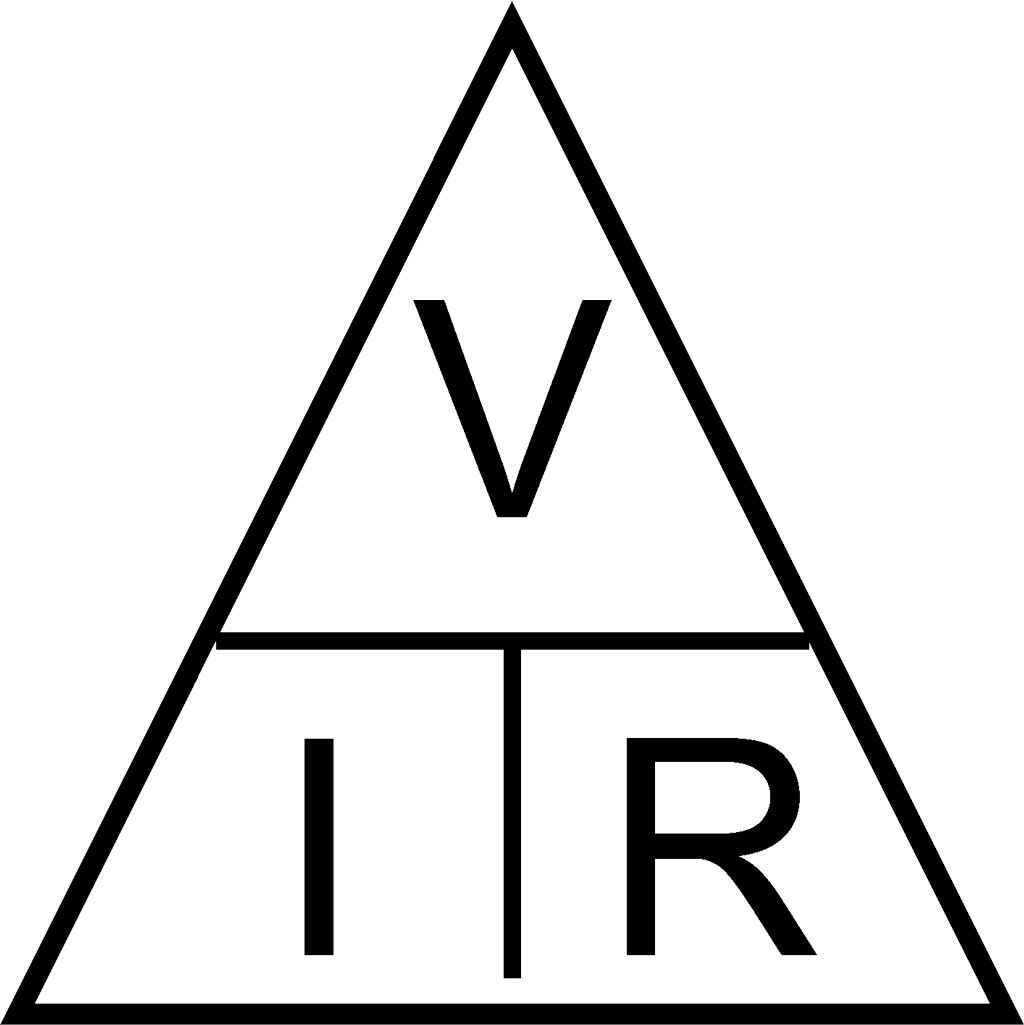(1) I = V / R
(2) V = I * R
(3) R = V / I

Where:
I is the current in amps,
V is the voltage in Volts, and
R is the resistance in Ohms.

The interchangeability of the equation may be represented by a triangle, where V (voltage) is placed on the top section, the I (current) is placed to the left section,
and the R (resistance) is placed to the right.
And now a good trick to remember easily the mathematic operations...
The line that divides the left and right sections indicate multiplication, and the divider between the top and bottom sections indicates division (hence the division bar).
So... if you want to find voltage, then cover V with your finger, and you are left with I and R.
As we said above, I and R which are in the same level, you have to multiply them, (V = I x R).
If you want to find current, then cover I with your finger, and you are left with V and R.
As we also said above, since V and R are in different levels, then you must divide them (I = V / R).
If you want to find resistance, then cover R with your finger. You should be left with V and I.
Finally, since V and I are in different levels, you must divide them, (R = V / I).

## Ohm's Law examples

We can now make some examples to see how this law works, on a simple circuit i drew below.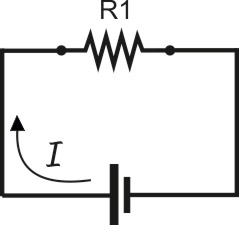Ok lets's say we have a power source of 5V
and a resistor value of R1 500Ohms
We want to calculate how much currnet will pass through the resistor....

Solving for... ( I ) We have I = V / R = 5 / 500 = 0.01A or 10mANow let's try it in a different approach...
suppose we know the power source of 5V and the current of 10mA
We want to calculate how much is the resistance of the resistor....

Solving for... ( R ) We have R = V / I = 5 / 0.01 = 500OhmsAnd finally, this time we are looking for the voltage.
We know the current that is 10mA and the resistance of R1 500Ohms

Solving for... ( V ) We have V = I x R = 0.01 x 500 = 5V

## Electrical power (Watt) The power triangle...

Electrical Power, (P) is the amount of energy that is absorbed or produced within a circuit.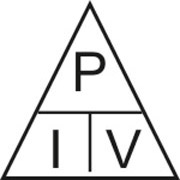A source of energy (Voltage) will produce or deliver power while the connected load absorb it.
Light bulbs for example, absorb power and convert it into heat and light.
Power is the amount of voltage multiplied by the current, and the unit of measurement is the Watt (W).

Now again, three equivalent expressions using of Ohm's law are used interchangeably:

(1) P = I * V
(2) I = P / V
(3) V = P / I

Where:
P is the power in watts,
I is the current in amps,
V is the voltage in Volts, and

The interchangeability of the equation represented on the triangle, can be solved as the above example that was given before.
It is worth mention another two types for finding Power....
Including Ohms law to this equation, we can create two more equations...

P = U x I
U = I x R
-------------
P = I2 x R (4)

And:

P = I x V
I = V / R
-------------
P = V2 / R (5)

## Electrical power (Watt) examples...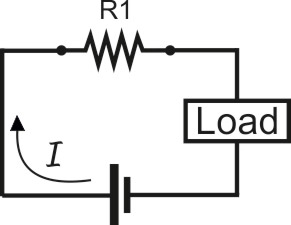Now suppose we have this circuit, and we know that values are same as above...   Source: 5V  /  Current: 0.01A /  R1:500Ohms
We can simply calculate how much power dissipation will be on R1 using

P = I * V = 0.01 * 5 = 0.05Watts

P = I2 * R = 0.0001 * 500 = 0.05Watts

P = V2 / R = 25 * 500 = 0.05Watts

### What is Energy ?

I believe that right now, we can make a brief report to Energy.
As we said above, Electrical Power, ( P ) is the amount of energy that is absorbed or produced within a circuit.

( Taken from wikipedia )
‟ In physics, energy is a property of objects which can be transferred to other objects or converted into different forms, but cannot be created or destroyed.

It is becoming very clear why resistors and other components heat up.....
A resistor transforms electric energy into heat. So the above circuit transforms 0.05 Watt (joules) of electric energy into heat every second.

This energy is called Power Loss and it's undesirable but also unavoidable. Too much power loss = Too much heat on a component.
But even when energy transforms, exploiting energy for the main goal of a component, there'll still be losses to other forms of energy.
For example: LEDs, motors, lamps etc, will still produce heat as a byproduct of their other energy transfers.
Remember... for each component there is a maximum power dissipation rating that they can dissipate.
Keeping these values on low operating ratings, you'll avoid the destruction of the component.